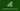# Pipe function in Javascript

Home/Pipe functions in Javascript

Nicola Capovilla - Aug 03, 2020

#Javascript#Pipe#Functions#FunctionalProgramming

## What is a pipe function?

The concept of pipe can often be interpreted in different ways, depending on context.
This is the definition of pipe based on the Linux's article: Pipes: A Brief Introduction.

A pipe is a form of redirection that is used in Linux and other Unix-like operating systems to send the output of one program to another program for further processing.

And:

Pipes are used to create what can be visualized as a pipeline of commands, which is a temporary direct connection between two or more simple programs. This connection makes possible the performance of some highly specialized task that none of the constituent programs could perform by themselves. A command is merely an instruction provided by a user telling a computer to do something, such as launch a program. The command line programs that do the further processing are referred to as filters.

We can define pipe function:

A pipe function is a function that accepts a series of functions, which process an input parameter and return a output which will be the input for the next function.

### Functional programming

The pipe function concept is related functional programming.
Functional programming is a programming paradigm where programs are constructed by applying and composing functions.

The goal is to compose pure functions avoiding shared state, mutable data, and side-effects. Functional programming is declarative rather than imperative which change the state of the program.

In Javascript there are several functions for functional programming, including reduce, which we are going to see later.

## Implementing a pipe function

We know that a pipe function take a series of functions and each function execute a series of operation based on the result of the previous function. We can start with a simple problem and evolve our pipe function step by step:

Given a number in input, first, add 2 and then multiply it by 2.

The result expression is:

``(n + 2) * 2``

Following the paradigm of functional programming awe would therefore have two atomic functions:

``````// Sum 2 to n
const sumTwo = n => {
return n + 2;
}

// Multiply 2 to n
const multiplyTwo = n => {
return n * 2;
}``````
• sumTwo: takes a number as input and returns the sum of the number with 2;
• multiplyTwo: takes a number as input and returns the multiplication of the number by 2;

The result:

``console.log(multiplyTwo(sumTwo(1))); // 6``

Generalize the result: encapsulating in a pipe function.

``````const pipe = (funA, funB) => (arg) => funB(funA(arg));
const result = pipe(sumTwo, multiplyTwo)(1);
console.log(result); // 6``````

## Reduce: refactor the pipe function

Last example, however, is limited only to pipe function of 2 functions, while, as previously explained, the objective is to accept N functions. Following the pipe function made in the previous chapter, the resulting implementation would be something like:

``````const pipe = (funA, funB, funC, ... , funN) => (arg) => {
funN( ... funC(funB(funA(arg))));
}``````

Now we can use Javascript's method Reduce, that executes a reducer function (that you provide) on each element of the array, resulting in single output value.

So we obtain our Pipe Function:

``````const _reduced = (f, g) => (arg) => g(f(arg));
const pipe = (...fns) => fns.reduce(_reduced);

// Example
const res = pipe(
sumTwo,
multiplyTwo,
moduleByTwo,
)(1)

console.log(res); // 0``````

### Explanation of reduce

The reducer function takes four arguments:

• Accumulator (acc)
• Current Value (cur)
• Current Index (idx)
• Source Array (src)

Your reducer function's returned value is assigned to the accumulator, whose value is remembered across each iteration throughout the array, and ultimately becomes the final, single resulting value.

``````# Index: 0
_reduced = sumTwo(arg)

# Index: 1
_reduced = (_reduced, multiplyTwo) => multiplyTwo(_reduced) -> multiplyTwo(sumTwo(arg))

# Index: 2
_reduced = (_reduced, moduleByTwo) => moduleByTwo(_reduced) -> moduleByTwo(multiplyTwo(sumTwo(arg)))

res = moduleByTwo(multiplyTwo(sumTwo(arg)))(1)``````

## Validation pipeline: pipe function without args

In some cases we need to perform a series of functions without worrying about the result of the previous function. A use case could be the validation of an element.
An element need to pass a series of functions that verify its validity (otherwise they throw an exception). We don't know if these functions modify the element passed and it could be useful to always use the first value, consequently we are going to modify the reduced in this way:

``````const validationPipe = (...fns) => (...args) => fns.reduce((res, func) => func(...args), ...args);

// Example
try {
pipe(
isNumber,
isGreaterThan10,
isAMultipleOf2,
)(12)

// Valid

} catch (e) {
// Invalid
}``````

## Next Step: The pipeline operator "|>" (experimental)

Recently Javascript introduce the experimental pipeline operator (|>). The MDN definition:

The experimental pipeline operator |> (currently at stage 1) pipes the value of an expression into a function.

This operator allows to write function like this:

``let url = decodeURI("%21");``

In:

``let url = "%21" |> decodeURI;``

So, we can obtain:

``````// Nested function
const res = moduleByTwo(multiplyTwo(sumTwo(arg)))(1);

// Pipe function
const res = pipe(
sumTwo,
multiplyTwo,
moduleByTwo,
)(1);

// Pipeline operator
const res = 1 |> sumTwo |> multiplyTwo |> moduleByTwo;``````

## Conclusions

These are some possible solutions to develop your own pipe function and follow the paradigms of functional programming.

The source code of the proposed solution you can see on this Github Repository.

Your support and feedback means a lot for us.StoriesMedium

GithubCodepen

## FollowItaliano